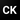Chandan Kumar

October 12, 2020

There are several methods for weight update available. These are called optimizers. Gradient descent optimization algorithm is one of these.

## Definition

• It is an optimization algorithm which finds and updates best values for the parameters of a mathematical function/model which minimizes it’s loss function (reduces error)
• To find a local minimum of a function, gradient descent takes steps proportional to the negative of the gradient of the function at the current point From our previous notes on Backpropagation $w_x^{new} = w_x - \eta (\frac {\delta Error} {\delta w_x})$

$$a^2 + b^2 = c^2$$

To update the $w_x$ , we take the current $w_x$ and subtract the partial derivative of the error function w.r.t. $w_x$ We also multiply the derivative of the error function with a number $\eta$ (usually small like 0.001) called learning rate to control the steps.

• Another example: Suppose we have a Linear regression model for a problem and using MSE (Mean squared error) as a loss function to calculate the error.

The equation of a linear regression model is: $y = mx + c$ where x is the input values, m & c are parameters of the equation

After prediction of values on the training dataset, the loss function MSE will calculate the error. Now job of Gradient Descent algorithm comes into action. It reduces the MSE by finding the best values for parameter m & c on the given dataset as values of m & c directly impacts the predictions done by the linear regression equation.

• Like linear regression model mentioned above, Gradient descent optimizes other Machine learning algorithms used during training.
• Always scale the data as Gradient descent converges faster if all the features are at same scale.
• Example of Gradient descent optimization algorithms:
• Momentum
• RMSProp

• It processes all the training data at once.
• In real-life ML problems, training data can be very large which will not fit in the memory at once so it is not preferred.

• It picks one instance of input data randomly (hence the name stochastic) and processes it at each iteration.
• This is faster as it consumes less memory, but number of iterations required increases which also becomes overhead to performance.
• Also, as it picks instances randomly, there is a lot of fluctuation while reaching to global minima.
• Always shuffle the training data at the beginning of each epoch. If not shuffled, SGD might pick similar instances on each epoch and will end up not reaching the global minima - It will work on less variations of input data, so obviously right! 🙂